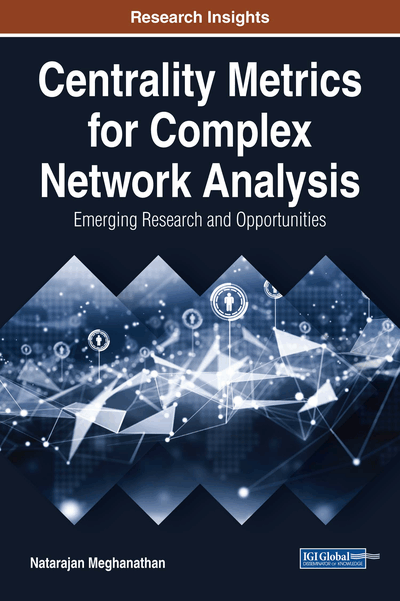# Temporal Variation of the Node Centrality Metrics for Scale-Free Networks

Copyright: © 2018 |Pages: 20
DOI: 10.4018/978-1-5225-3802-8.ch004

## Abstract

Scale-free networks are a type of complex networks in which the degree distribution of the nodes is according to the power law. In this chapter, the author uses the widely studied Barabasi-Albert (BA) model to simulate the evolution of scale-free networks and study the temporal variation of degree centrality, eigenvector centrality, closeness centrality, and betweenness centrality of the nodes during the evolution of a scale-free network according to the BA model. The model works by adding new nodes to the network, one at a time, with the new node connected to m of the currently existing nodes. Accordingly, nodes that have been in the network for a longer time have greater chances of acquiring more links and hence a larger degree centrality. While the degree centrality of the nodes has been observed to show a concave down pattern of increase with time, the temporal (time) variation of the other centrality measures has not been analyzed until now.
Chapter Preview
Top

## 1. Introduction

Real-world networks, ranging from biological networks to the Internet, exhibit complex relationships among the nodes. The complexity is significantly reduced when we model the real-world network as a graph of vertices (representing the nodes) and edges (representing the vertices). There exists well-known theoretical models to generate synthetic graphs whose degree distribution matches with that of the real-world network graphs. Some of the well-known graph theoretical models are the random graph model (Erdos & Renyi, 1959), scale-free model (Barabasi & Albert, 1999) and small-world network model (Watts & Strogatz, 1998). The degree distribution of the random network graphs follows the Poisson model (Erdos & Renyi, 1959) and real-world networks such as the US road network, citation networks, scientific collaboration networks and etc also exhibit a Poisson model (Bornholdt & Schuster, 2003; bell-shaped pattern whose width is a function of the standard deviation of the distribution) for the degree distribution of the vertices. The degree distribution of the scale-free network graphs (Barabasi & Albert, 1999) follows the Power law model and real-world networks such as the World Wide Web, Airline networks, Social networks, Protein-protein interaction networks and etc. exhibit a Power law model for the degree distribution of the vertices (Caldarelli, 2007; a distribution in which the probability for finding nodes with larger degree decreases at a faster rate but the distribution has a long tail).

Our focus of study in this chapter will be on the Barabsi-Albert (BA) model (Barabasi & Albert, 1999) for generating scale-free networks. We seek to analyze the evolution of a scale-free network (under the BA model) over time with respect to the distribution of the centrality metrics (referred to as temporal variation of the centrality metrics). Most of the results currently available (Barabasi & Albert, 1999) in this regard pertains to the degree centrality metric and we briefly review these below: Scale-free networks are characteristic of having a few nodes (but appreciable number of nodes) that have a significantly larger degree than the rest of the nodes in the network. Such nodes are called the hub nodes. Under the BA model for generating scale-free networks, the probability for an existing node to get a new link is proportional to its degree; i.e., new nodes (at the time of joining the network) are more likely to prefer being linked to nodes that have a larger degree (rather nodes with smaller degree). As a result of this “preferential attachment” phenomenon, nodes that joined the network during the earlier stages of evolution exhibited a relatively faster increase in their degree compared to nodes that joined the network during the later stages. Thus, the hub nodes of a scale-free network that evolved under the BA model are those nodes that joined the network much earlier and the nodes that joined at a later time are less likely to become hub nodes.

## Complete Chapter List

Search this Book:
Reset